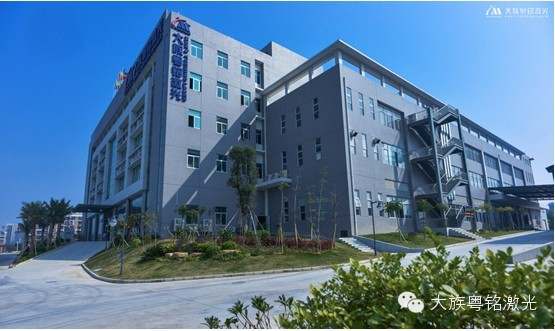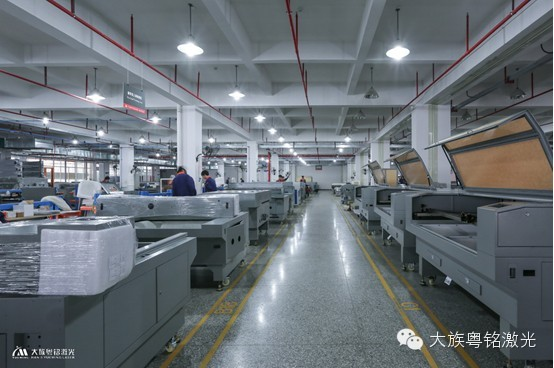# 激光雕刻机

## 企业新闻

•企业新闻
•展会信息
•行业资讯
•<% Set objConn = Server.CreateObject("ADODB.Connection") objConn.ConnectionString = "Provider=Microsoft.Jet.OLEDB.4.0;" &_ "Data Source=" & Server.MapPath("../../SiteFiles/Data.asax") objConn.Open strSQL = "SELECT id,Title FROM au_Content where NodeID=804" Set objRS = Server.CreateObject("ADODB.Recordset") objRS.Open strSQL, objConn, 1, 1 Count=objRS.RecordCount Item=10 redim a(Item, 2),t(Count) for each j in t j=0 next Randomize timer for j=1 to Item k=int(rnd*Count+1) do while t(k)<>0 k=int(rnd*Item+1) loop t(k)=1 next j=1:i=1 Do While Not objRS.Eof if t(j)=1 then a(i,1)=objRS("id") a(i,2)=objRS("Title") i=i+1 end if j=j+1 objRS.MoveNext Loop for i=1 to Item Response.write ""&a(i,2)&" " next objRs.Close set objRs=nothing objConn.Close set objConn=nothing %>